# VMO-space

Jump to: navigation, search

space of functions of vanishing mean oscillation

The class of functions of vanishing mean oscillation on, denoted by, is the subclass of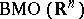consisting of the functionswith the property that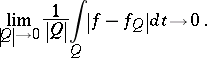Here,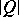denotes the volume of the ballanddenotes the mean ofover(see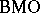-space). As with,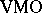can be defined for spaces of homogeneous type.

Some properties ofare as follows (see also [a1], [a2], [a3]). Bounded, uniformly continuous functions are in(cf. Uniform continuity), andcan be obtained as the-closure of the continuous functions that vanish at infinity. The Hilbert transform of a bounded, uniformly continuous function onis in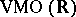.is the dual of the Hardy space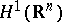(cf. also Hardy spaces).appears in the theory of Douglas algebras: Letbe the boundary of the unit disc in. Let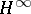denote the subspace ofconsisting of the boundary values of bounded holomorphic functions (cf. Analytic function) on the unit disc and letdenote the set of continuous functions on. Put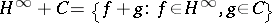.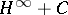is a closed subalgebra ofand the simplest example of a Douglas algebra. Its largest self-adjoint subalgebra,, equals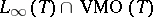, [a2], [a3].